# # Wavefunction Collapse

An algorithm for constraint satisfaction, commonly applied to generative image synthesis from an input image.

See description with pictures at: https://robertheaton.com/2018/12/17/wavefunction-collapse-algorithm/

## # 1 Tile Extraction

Suppose tiles are D×D pixels. For simplicity assume D is odd so each tile has a unique center pixel. With 3×3 tile size, a 6×6 image will have 4×4 = 16 overlapping subtiles. Form a unique set from all the subtiles in the image (discard repeats, but count them to give a probability distribution) and label each with a distinct label (consecutive small integers are convenient).

## # 2 Tile Transitions

Let N be the radius of influence. For odd D this will be N = (D + 1)/2. For each tile, and each (i,j) in (-N,-N) to (N,N) inclusive, scan the image to build an array of sets of allowed labels for each tile and offset.

## # 3 Background

Initialize the output tiles to a grid with each cell containing a set of all possible labels. In C++, std::vector<bool> may be used for compact std::set<int>.

## # 4 Collapse

If all cells have entropy 0, then the algorithm has finished successfully: each cell has only one tile possibility.

Otherwise, pick one of the the lowest positive entropy output cells at random, and pick one of its possibilities at random (weighted by the tile probability distribution), and collapse its possibilities to only the chosen possibility.

Then propagate constraints to neighbours (recursively).

## # 5 Constraint Propagation

For a cell in the output grid, consider its neighbours with offsets (-N,-N) to (N,N) inclusive.

For each neighbour, intersect its possibilities with the union of the allowed labels for the offset (i,j) for each possibility of the original cell.

If the neighbour possibilities changed, propagate its constraints too, and so on. In this way the possibility sets are reduced in a wavefront that moves across the image.

If any possibility set becomes empty, a contradiction occured: easiest way to recover is to start over from the beginning.

## # 6 Variations

If instead of extracting allowed tile transitions from the input, one extracts all possible tile transitions from the tile set by seeing how the tiles can overlap, images have a different character.

This may or may not be desirable.

## # 7 Examples

### # 7.1 Checkerboard

For a black and white checkerboard (1×1 cells), and 3×3 tiles, there will be two possible subtiles in the image (if it is large enough). Label them “0” for black top left pixel, “1” for white top left pixel. D = 3 so N = 2. The set of allowed neighbours of “0” will be {“0”} for offsets (i,j) where i+j is even and {“1”} for offsets (i,j) where i+j is odd. Similarly, allowed neighbours of “0” will be {“1”} for offsets (i,j) where i+j is even and {“0”} for offsets (i,j) where i+j is odd.

These sets are all singletons, so collapsing any single tile will lead to ripples collapsing all tiles in the image.

### # 7.2 Checkerboards

Input input: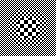3×3 tiles.

#### # 7.2.1 Transitions From Image

52 tiles, 2984 transitions.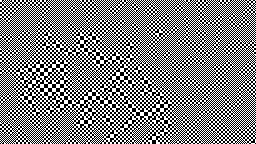#### # 7.2.2 Transitions from Tiles

Exhibits unexpected textures.

52 tiles, 13872 transitions.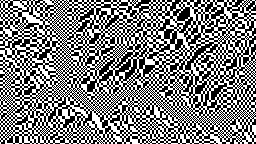### # 7.3 Flowers

Input image: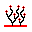3×3 tiles.

#### # 7.3.1 Transitions From Image

Exhibits verbatim copying.

120 tiles, 6106 transitions.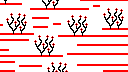#### # 7.3.2 Transitions from Tiles

120 tiles, 62678 transitions.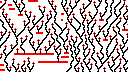## # 8 Videos

One frame is emitted each time a cell is collapsed.

## # 9 Code

git clone https://code.mathr.co.uk/wavefunction-collapse.git